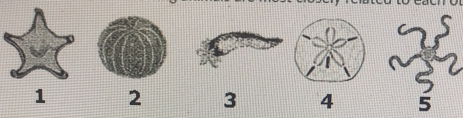# Problem: Which two of the following animals are most closely related to each other?a. 1 and 4b. 1 and 5c. 2 and 3d. 3 and 5e. 2 and 4

###### FREE Expert Solution

1 and 5: radial symmetry, display pentamerism arranged in a central axis###### Problem Details

Which two of the following animals are most closely related to each other?a. 1 and 4

b. 1 and 5

c. 2 and 3

d. 3 and 5

e. 2 and 4